### Vol 17, No 5 (2017) / Xing

The penetrating depth analysis of Lunar Penetrating Radar onboard Chang’e-3 rover

# The penetrating depth analysis of Lunar Penetrating Radar onboard Chang’e-3 rover

Xing Shu-Guo1, 2, , Su Yan1, Feng Jian-Qing1, Dai Shun1, Xiao Yuan1, Ding Chun-Yu1, Li Chun-Lai1

National Astronomical Observatories, Chinese Academy of Sciences, Beijing 100012, China
University of Chinese Academy of Sciences, Beijing 100049, China

† Corresponding author. E-mail: suyan@nao.cas.cn

Abstract: Abstract

Lunar Penetrating Radar (LPR) has successfully been used to acquire a large amount of scientific data during its in-situ detection. The analysis of penetrating depth can help to determine whether the target is within the effective detection range and contribute to distinguishing useful echoes from noise. First, this study introduces two traditional methods, both based on a radar transmission equation, to calculate the penetrating depth. The only difference between the two methods is that the first method adopts system calibration parameters given in the calibration report and the second one uses high-voltage-off radar data. However, some prior knowledge and assumptions are needed in the radar equation and the accuracy of assumptions will directly influence the final results. Therefore, a new method termed the Correlation Coefficient Method (CCM) is provided in this study, which is only based on radar data without any a priori assumptions. The CCM can obtain the penetrating depth according to the different correlation between reflected echoes and noise. To be exact, there is a strong correlation in the useful reflected echoes and a random correlation in the noise between adjacent data traces. In addition, this method can acquire a variable penetrating depth along the profile of the rover, but only one single depth value can be obtained from traditional methods. Through a simulation, the CCM has been verified as an effective method to obtain penetration depth. The comparisons and analysis of the calculation results of these three methods are also implemented in this study. Finally, results show that the ultimate penetrating depth of Channel 1 and the estimated penetrating depth of Channel 2 range from 136.9 m to 165.5 m () and from 13.0 m to 17.5 m (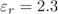), respectively.

## 1 Introduction

The Chang’e-3 satellite was successfully launched from the Xichang satellite launching center on 2013 December 2 and landed in northern Mare Imbrium after 12 days. With successful separation of lander and rover, the Yutu lunar rover started its observation mission. Lunar Penetrating Radar (LPR), as one of the important scientific payloads on the Yutu lunar rover, obtained a large amount of scientific data.

LPR is a kind of nanosecond radar operating in the time domain, with separate transmitting and receiving antennae. The ultra-broadband nanosecond pulse generated by the transmitting antenna of LPR propagates into lunar regolith and crustal rock. If the pulse encounters an uneven layer or the interface of different media, it will be reflected and scattered back to the radar. Through data processing, analysis and inversion, the thickness and distribution of lunar regolith as well as the geological structure of the lunar subsurface along the rover’s path can be obtained. In the LPR system, two detection channels are designed. Channel 1 has a center frequency of 60 MHz with a band of 40–80 MHz, while Channel 2 has a center frequency of 500 MHz with a band of 250–750 MHz. With a depth resolution of meter-scale, Channel 1 aims to detect the structure of the shallow lunar crust. Also, with a depth resolution of less than 30 centimeters, Channel 2 is used to detect the thickness and structure of lunar regolith (Fang et al. ).

The LPR detection on the Moon can be divided into three stages. The first stage was during the first Moon day after the rover landed, on which the rover moved from navigation point N0101 to N0108. Some useful parameters, such as the system gain, time window and attenuation settings, were tested and eventually confirmed to ensure the LPR worked optimally for detection. The second stage was during the second Moon day, on which the rover moved from point N0201 to N0209. Lots of detection data were obtained in this stage. In the third stage, which corresponded to the third Moon day and later time, some detection data were obtained in the permanent point (N0209) under different settings, such as radar high-voltage-off, radar high-voltage-on and different attenuation values.

A number of investigators have done scientific researches using the LPR data and initial results were reported (Su et al. , Zhang et al. , Dai et al. ). However, no research about the penetrating depth of LPR has been carried out in these studies. The penetrating depth is very important. On one hand, it can be used to determine whether the target is within the effective detection range. On the other hand, it may help to distinguish useful echoes from noise, which will eventually contribute to noise suppression and layer recognition (Davis & Annan ). Moreover, complex and heterogeneous lunar media (Heiken et al. ) will complicate reflected echoes, so it is particularly important to find the boundary between reflected echoes and noise.

As an electromagnetic signal propagates in a medium, it will attenuate. Actually, there will be a reflected signal if any different impedance exists in different media, and the reflected signal will be received by the receiving antenna, so the penetrating depth is theoretically infinite. However, existing noise and the restriction of minimum detection power in the LPR system make the penetrating depth finite. In other words, when the reflected signal is less than the minimum detection power or submerged in noise, it is very difficult to detect. Although a signal submerged in noise can be detected with some methods (Tugnait , Gelchinsky & Shtivelman , Coppens ), mostly certain conditions are required. In this study, the penetrating depth means the boundary between the last useful reflected echoes and noise.

In this paper, two methods based on a radar transmission equation are introduced in Section 2, in which the first method adopts the system calibration parameters and the second one uses high-voltage-off radar data. Then, a new method termed the Correlation Coefficient Method (CCM) is proposed in Section 3 and a simulation process has been conducted to test the validity of this new method. In Section 4, the CCM is used for the Chang’e-3 LPR radar data. A comparison of the results of these three methods is described in Section 5, while the conclusions are drawn in Section 6.

## 2 The Methods Based on Radar Equation

In this section, two methods based on the radar equation are introduced to calculate the penetrating depth. First, the method with system calibration parameters is described and the penetrating depths of two channels (60 MHz and 500 MHz) are estimated. Then, using high-voltage-off data, the minimum detection power is modified and new penetrating depths of the two channels are obtained correspondingly.

## 2.1 Estimation of Penetrating Depth with System Calibration Parameters

According to the system calibration parameters (system gain and minimum detection power) and the radar equation, this method may estimate the penetrating depth in theory. As shown in Figure 1, the transmitting antenna radiates an electromagnetic pulse signal with transmitting power P t . When the signal transmits in the media, there is attenuation in the whole transmission path. ζ 1 means the penetrating attenuation from the air to medium 1, and ζ 2 means the penetrating attenuation from medium 1 to air. R is the penetrating depth. σ is the radio scattering sectional area. When the reflected echo power is equal to the minimum detection power, the maximum penetrating depth can be calculated.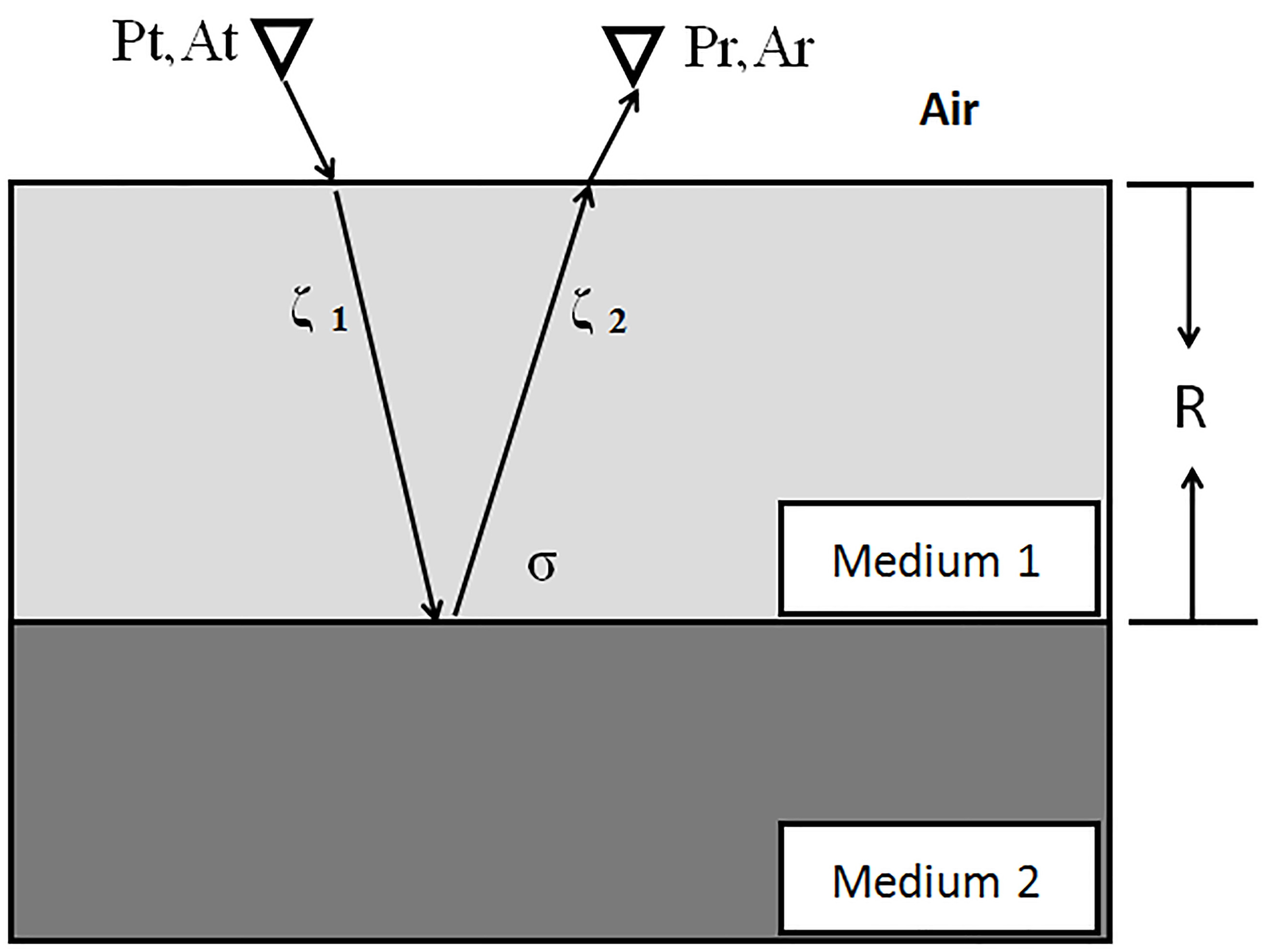Fig. 1 Model for the penetrating depth calculation based on the radar equation.

Using the traditional radar equation (Ulaby et al. ), we can get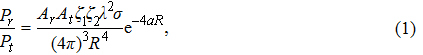where P r means the received power, P t means the transmitted power, A r is the receiving antenna gain and A t is the transmitting antenna gain. λ is the wavelength in the medium.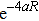means attenuation in the medium. a means the attenuation constant.

The traditional radar equation can also be written in the form of system gain, which is shown as follows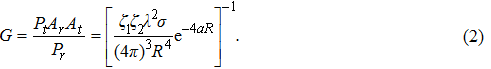G is the system gain. The maximum detection depth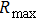can be obtained when P r equals the minimum detection power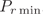. This equation can further be expressed as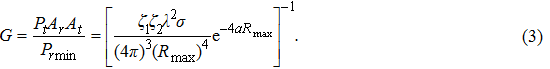In Equation (3), attenuation constant a is calculated by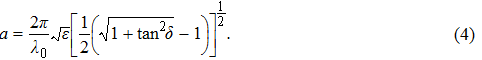The radio scattering sectional area is calculated by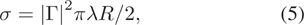where Γ is reflection coefficient.

The penetrating attenuation between two media under vertical incidence can be evaluated using the following equations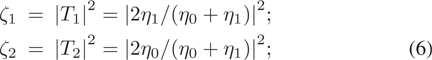and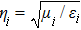, where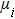and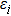mean permeability and permittivity respectively.

The system gain and the minimum detection power of Channel 1 are 152 dB and –124 dB, while they are 133.3 dB and –118 dB respectively for Channel 2, according to the calibration report (Zhang et al. ). In order to use the above radar equation, there are also two parts we need to figure out. The first part is the lunar subsurface structure: with the assumption of a two layer structure (lunar regolith and crustal rock), for Channel 1 data, we only consider the attenuation of rock and the reflection coefficient between the rock and lunar regolith, while the attenuation of regolith and reflection coefficient between the lunar regolith and rock are considered for Channel 2 data. The second part is the dielectric property of the media, which contains the relative dielectric of lunar regolith, the relative dielectric of lunar crustal rock and the loss tangent of this area. Statistics and analysis of samples from Apollo 15–17 (Heiken et al. ) and inversion data from KAGUYA (Ono et al. ) suggest that the relative dielectric of lunar regolith is from 2.3 to 3.5, while the relative dielectric of lunar crustal rock is from 6.6 to 8.8. The Chang’e-3 landing site is a young lava flow in the northeastern part of Mare Imbrium where the extremely rich FeO and (FeO + TiO2) content has reached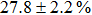(Ling et al. ). Using a two-dimensional formula derived from Apollo 15–17 samples by Heiken (Heiken et al. ), the loss tangent of this landing site ranges from 0.0117 to 0.0159.

Figure 2 indicates the relationship among the penetrating depth, loss tangent (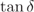) and relative dielectric constant ε r . The left is for Channel 1 (60 MHz) and the right is for Channel 2 (500 MHz). The figure shows that with a smaller dielectric constant and smaller loss tangent, the radar can reach a deeper penetrating depth, which agrees with reality. Therefore, with the central frequency of 60 MHz,of 0.0117 and a relative dielectric constant of 6.6, the maximum penetrating depth of Channel 1 is 152.4 m and the associated double time delay is 2610.4 ns, while the maximum penetrating depth of Channel 2 is 18.4 m and its double time delay is 185.5 ns (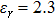,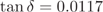,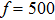MHz).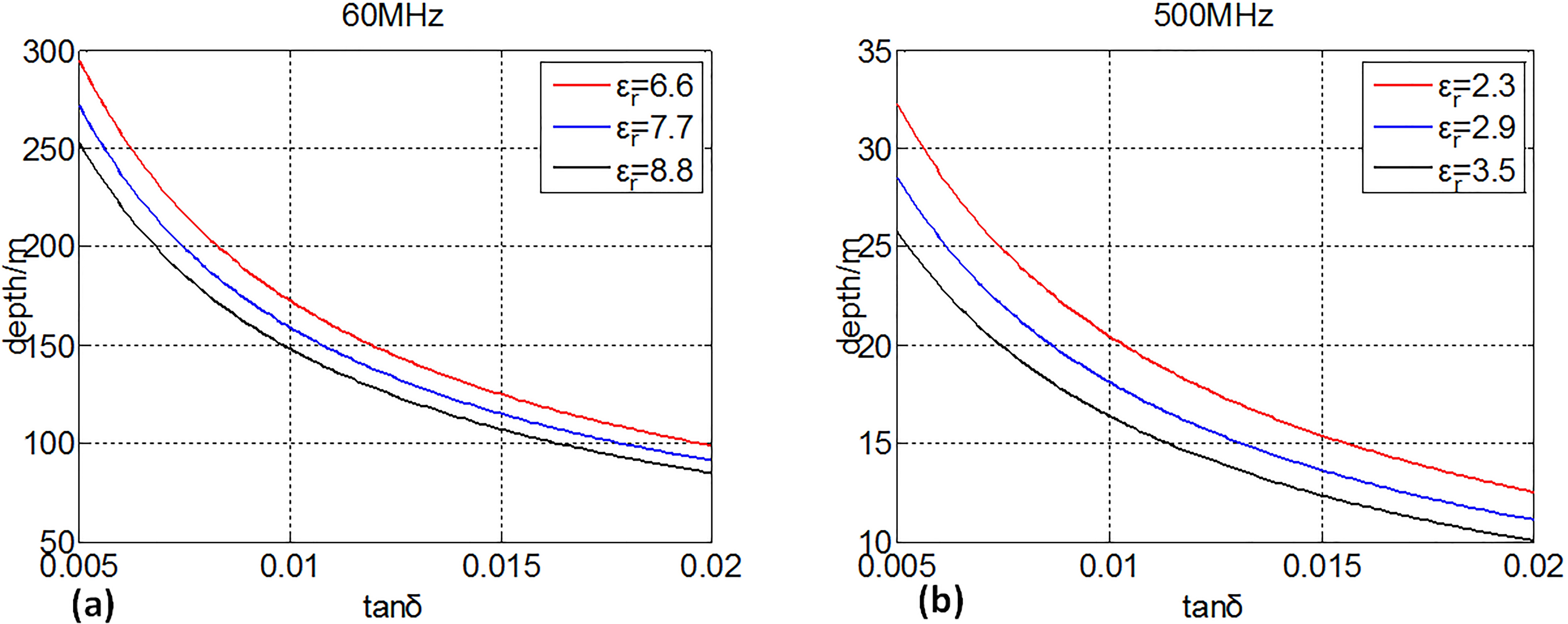Fig. 2 The penetrating depth of LPR; the left is for 60 MHz; the right is for 500 MHz.

## 2.2 Estimation of Penetrating Depth Using High-Voltage-Off Data

Considering that the noise of LPR data may be greater than the minimum detection power, if we still use the minimum detection power as the threshold of penetrating depth, it will be inaccurate. So in this section, high-voltage-off data from navigation point N0209 are used to calculate the noise floor. At the navigation point N0209, the high-voltage of the LPR transmitter was closed, which means that the LPR only received background noise. In the absence of strong interference introduced by the transmitting signal, the data are more accurate for identifying noise. After comparison of the noise floor with minimum detection power, the minimum detection power is replaced by the noise value. With the new minimum detection power and radar transmission equation in Section 2.1, the new penetrating depth is obtained.

The data acquired from 2014–02–15 T23:19:14 to 2014–02–15 T23:24:57 are chosen for estimation of penetrating depth. During this period, the LPR was working with the same settings as in the second stage. One trace of data from Channel 1 and Channel 2 is shown in Figures 3 and 4, respectively. The reason for the weak signal appearing at the beginning of the data is that even if the high-voltage is off, some fast edge signals will be produced by a microwave dynatron (12 V) and some weak signal coupling in an internal link still exists. When processing the Channel 1 data, we choose six different-size data blocks (with sample points 600–4000, 1500–4000, 2000–4000, 2500–4000, 3000–4000, and 3500–4000) in order to skip the weak and initial signal. The mean and standard deviation of each data block are calculated and shown in Table 1. The six selected data blocks of Channel 2 and the corresponding mean and standard deviation are listed in Table 2. The means of Channel 1 and 2 is approximately zero and the variation range of standard deviation in different blocks is less than 2%, which is less than the 5% bias of the transmitter pulse amplitude (Zhang et al. ). The result shows that in the selected blocks the noise is dominant and the method is effective for estimating noise.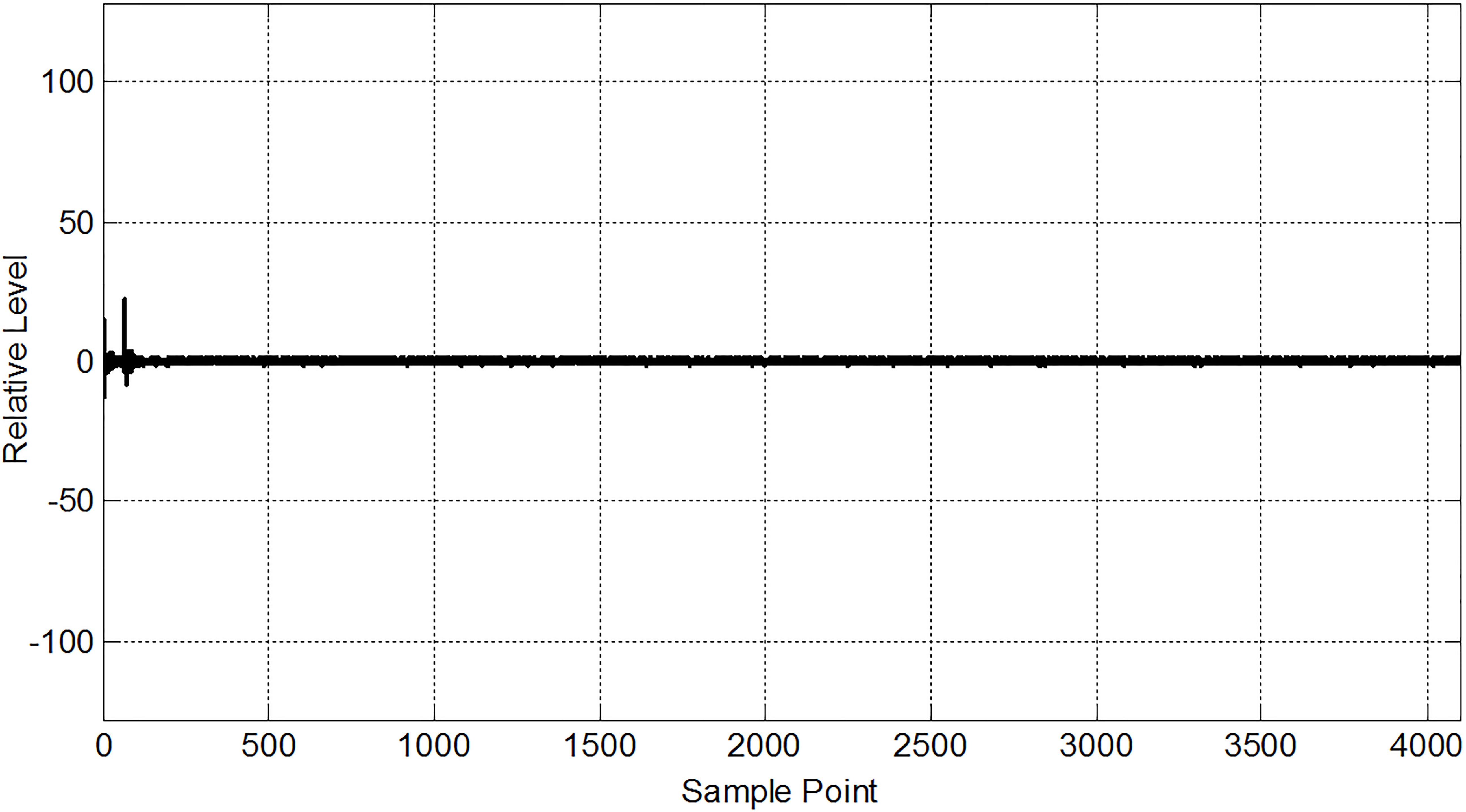Fig. 3 One trace of data representing high-voltage-off data from Channel 1.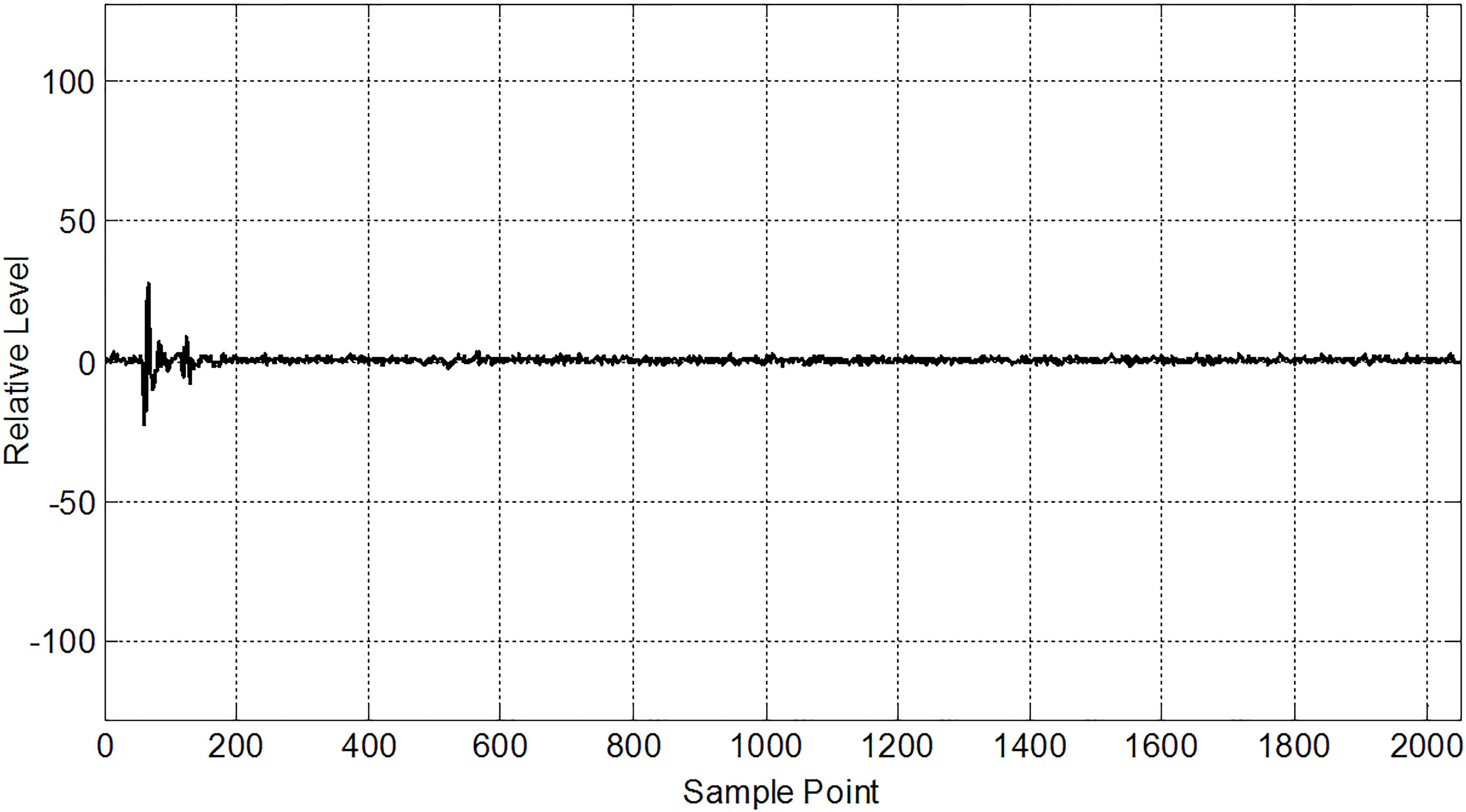Fig. 4 One trace data representing high-voltage-off data from Channel 2.
Sample Points 1000–4000 1500–4000 2000–4000 2500–4000 3000–4000 3500–4000
Standard Deviation 2.1265 2.1065 2.1387 2.1193 2.1239 2.0986
Mean 0.0012 0.0066 0.0000 0.0020 –0.0020 0.0136

Table 1 The Mean and Standard Deviation of Different Blocks from Channel 1

Sample Points 600–2000 800–2000 1000–2000 1200–2000 1400–2000 1600–2000
Standard Deviation 0.6981 0.6969 0.7038 0.6837 0.7036 0.7073
Mean 0.0051 0.0036 0.0138 0.0173 0.0194 0.0149

Table 2 The Mean and Standard Deviation of Different Blocks from Channel 2

The mean and the standard deviation of the noise floor associated with Channel 1 are 0 and 2.1, respectively. Taking three times the standard deviation and referring to the gain control method (Fang et al. ), the power of noise is –117.1 dB, which is greater than the minimum detection power in Channel 1 (–124 dB). Replacing the minimum detection power with –117.1 dB, Figure 5(a) shows the new penetrating depth curve for Channel 1. The figure shows the maximum penetrating depth of Channel 1 is 123.3 m and the double time delay is 2112.3 ns (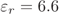,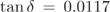,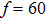MHz).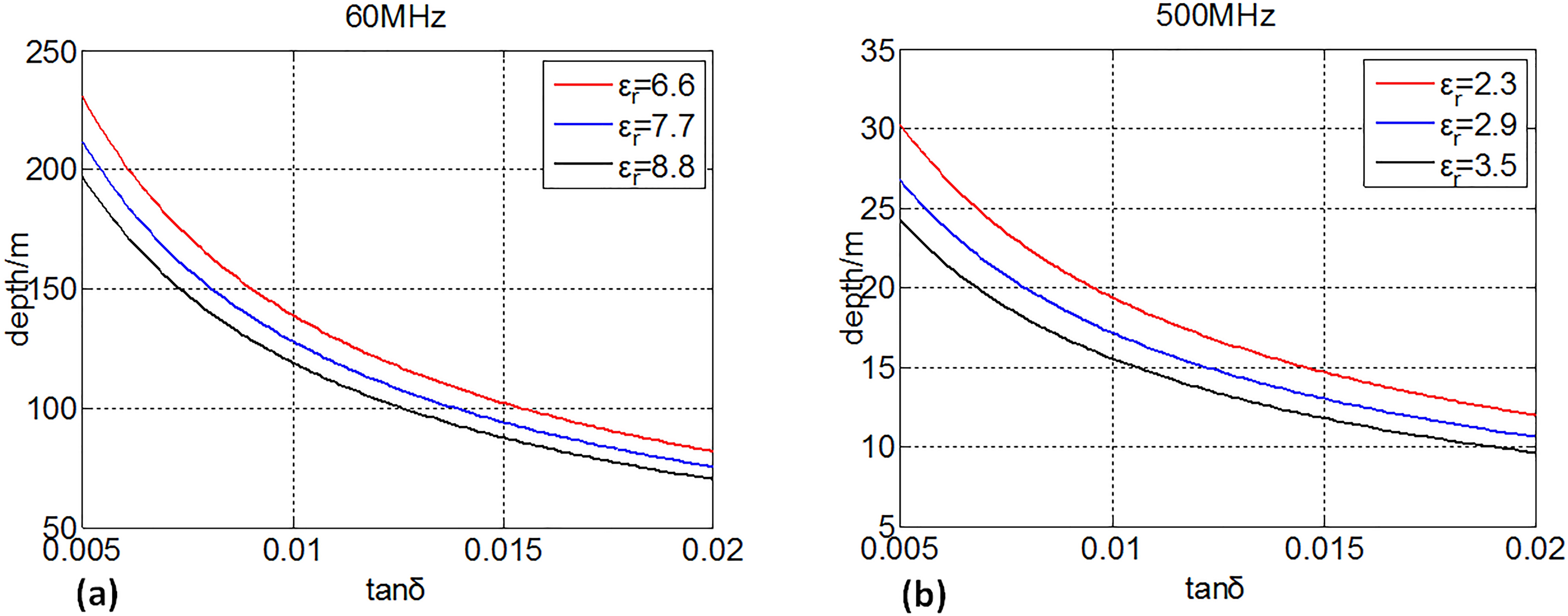Fig. 5 The penetrating depth of LPR; the left is for 60 MHz; the right is for 500 MHz.

The mean and the standard deviation of the noise floor for Channel 2 are 0 and 0.7, respectively. Taking three times the standard deviation, the power of noise is –115.8 dB, which is greater than the minimum detection power for Channel 2 (–118 dB). Based on the method in Section 2.1, replacing the minimum detection power with –115.8 dB, the new penetrating depth curve of Channel 2 is shown in Figure 5(b). The figure shows the maximum penetrating depth of Channel 2 is 17.4 m and the double time delay is 176.1 ns (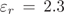,,MHz).

## 3 Correlation Coefficient Method

In Section 2, two methods based on the radar equation to calculate the penetrating depth need a priori assumptions. A new method termed CCM is introduced to estimate the depth, which is only based on measured radar data. The new method can avoid a priori assumptions and error produced by the difference between the ground calibration environment and the in-situ detection environment. Moreover, the new method can also show a distance-varying penetrating depth along the profile of the rover.

## 3.1 The Principle

The difference in correlation coefficients between reflected echoes and noise in the LPR data profile is used to estimate the penetrating depth of radar waves. The experiments demonstrate that reflected echoes of adjacent traces have a high correlation, namely high resemblance, but noises have random correlation.

Figure 6(a) and Figure 7(a) show one trace signal from Channel 1 and Channel 2 respectively. The correlation coefficients of each sample point calculated with the adjacent trace are shown in Figure 6(b) and Figure 7(b) respectively.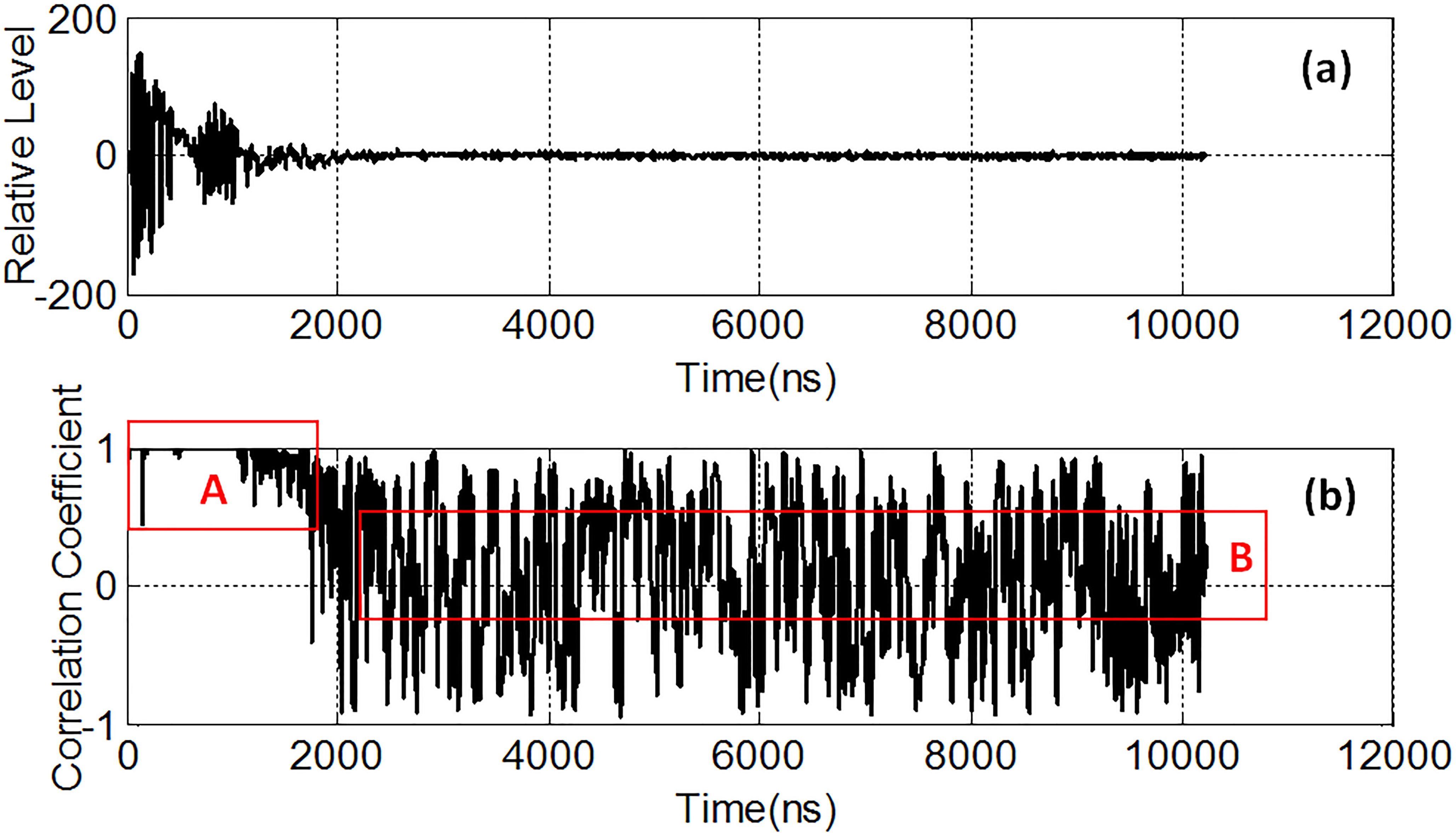Fig. 6 One trace signal from Channel 1 and the correlation coefficient of each sample point. (a) The original signal and (b) the correlation coefficient distribution.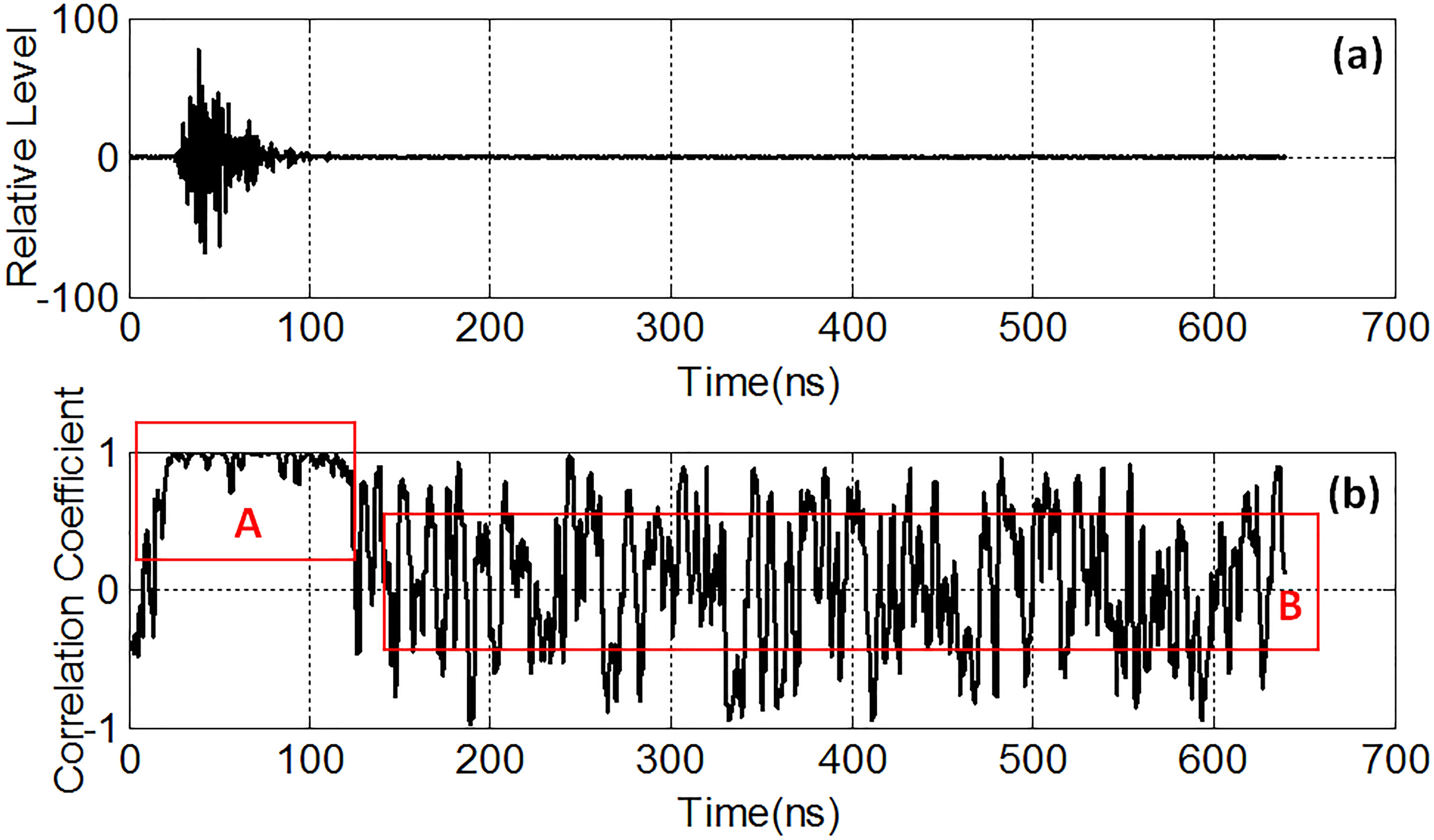Fig. 7 One trace signal from Channel 2 and the correlation coefficient of each sample point. (a) The original signal and (b) the correlation coefficient distribution.

Figure 6(b) and Figure 7(b) show that the trend in the correlation coefficient curve can be divided into two parts. The first part, denoted with A, shows the high correlation coefficient and illustrates that useful reflected echoes are dominant in this part. The second part, denoted with B, shows the random correlation coefficient without a continuous high correlation area, which illustrates that random noise is dominant in this part. The boundary point from high correlation to random correlation is the penetrating depth that we need to identify. Based on these characteristics, the detailed processing steps are as follows.

Let X be the original radar data matrix with a size of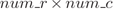, whereis the total number of samples of one trace and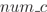is the total number of traces; C is the correlation coefficient matrix with a size of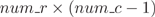; D is the cumulation-division matrix with a size of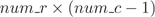.

Step 1. Select the sliding window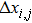. Once the sliding window is determined, the length of the following correlation window is decided correspondingly.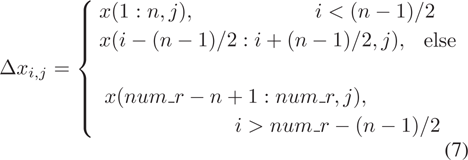where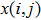is the value of the sample point of i at the j-th trace in matrix X, and n is the length of a sliding window, which should be larger than the width of a wavelet and always be an odd number.in Channel 1 and in Channel 2 are 4096 and 2048, respectively.

Step 2. Calculate the mean of the selected sliding window of trace j and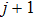;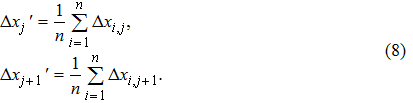Step 3. Calculate the correlation coefficient based on two adjacent trace data;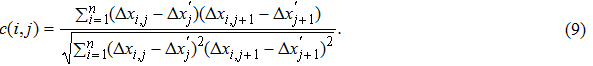Step 4. Repeat steps 1 to 3 from i= 1 to, j = 1 to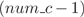to obtain correlation coefficient matrix C;

Step 5. Extract depth curve. First, obtain the cumulation-division matrix D. m is cumulation length, typically 200 is selected.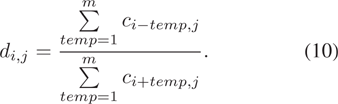Second, find the location of maximum value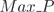in each column of D to extract the penetrating depth curve; the length ofis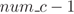;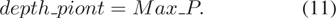Step 6. Compensate the correlation error. The final penetrating curve should subtract half the correlation interval.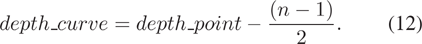Actually, in the correlation coefficient matrix C, the boundary between high correlation and random correlation is already clearly shown. The final curve is retrieved in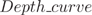. Because the correlation coefficient is calculated by a window which is not a signal point, when determining the boundary point, an ineluctable error might be introduced although error compensation was conducted in Step 6. However, the error is less than half the interval.

## 3.2 Simulation and Verification

In this section, a simulation is built to verify the validity of CCM.

Figure 8(a) is a typical radar profile with a time window of 48 ns and 60 traces. In order to generate a depth curve, we simulated a curve shown in Figure 8(b) and the curve is composed of 60 points. We combine Figure 8(a) with Figure 8(b) and add Gaussian noise (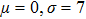) into it. The new profile is shown in Figure 8(c). The red line is the boundary depth between reflected echoes and noise. Above the red line it contains noise and reflected echoes, while only noise exists below the red line.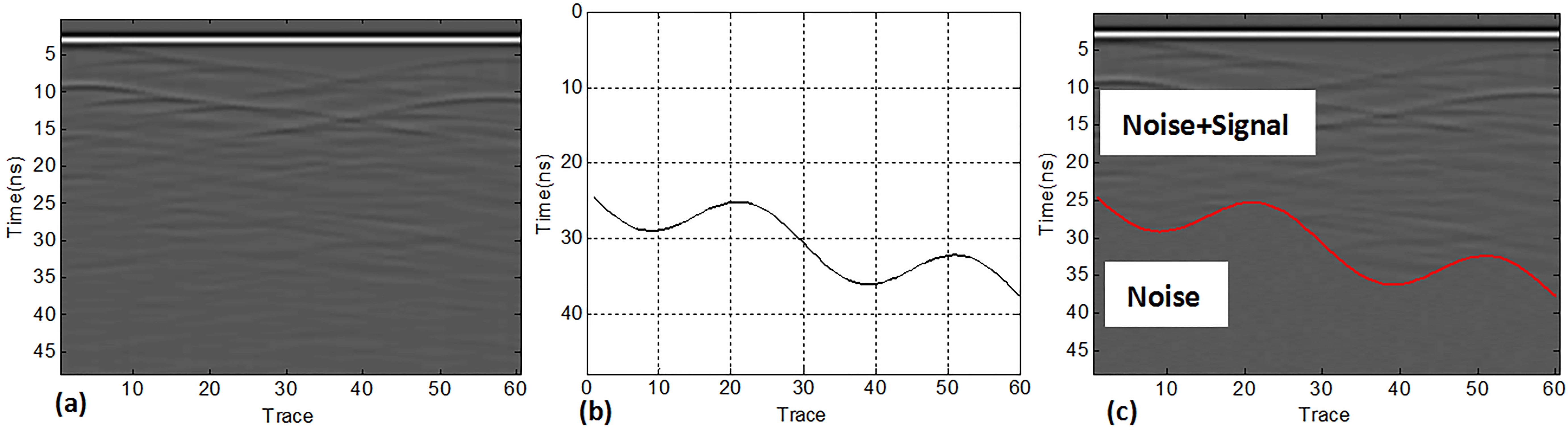Fig. 8 The established simulation model. (a) The typical radar profile; (b) the artificial depth curve; (c) in the radar profile with an artificial depth curve, noise is below the red line, and above the red line it contains noise and signal.

Based on CCM, firstly we obtained the correlation coefficient matrix shown in Figure 9(a). The length of interval (time window for CCM) we choose is one wavelet (5 ns). Figure 9(a) clearly shows the boundary line between the reflected echoes and noise. Secondly, we extracted the penetrating depth curve. A comparison between the estimated curve and the real depth curve is shown in Figure 9(b). Since the interval is 5 ns, the acceptable error is less than 2.5 ns. An error bar of ±2.5 ns is added to the estimated curve in Figure 9(b). The results reveal that the error between the estimated curve and the real depth curve is within the range of half a wavelet. Therefore, CCM is an effective method to acquire the boundary line between the reflected echoes and noise; the estimated depth error is less than half a wavelet.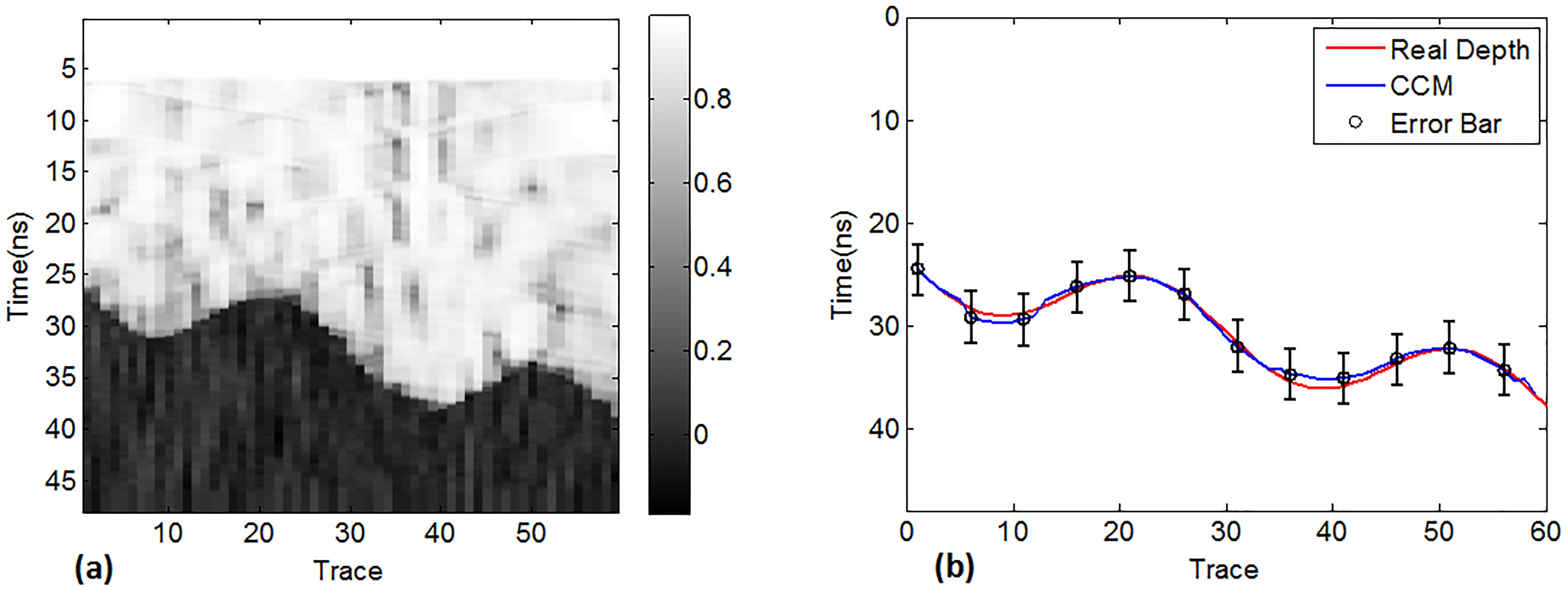Fig. 9 The correlation coefficient map and the estimated depth by CCM. (a) The correlation coefficient map; (b) the comparison between real depth and estimated depth by CCM.

## 4 Data Processing Based on Lunar data

The CCM was applied to process Chang’e-3 radar data. Data taken by Channel 1 from N0106 to N0207 are chosen, while data from N0105 to N0208 are selected for Channel 2. While operating at these navigation points, the radar worked with the same instrument parameters, thus we can splice these data together.

Based on the CCM (the sliding window n of 60 MHz data is 13 points and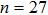@500 MHz, which is about equal to the width of a wavelet), the original data profile and correlation coefficient map of Channel 1 are shown in Figure 10, while the ones of Channel 2 are shown in Figure 11. The correlation coefficient maps demonstrate that there is a boundary line between the upper echoes and the lower noise.Fig. 10 The original radar profile and correlation coefficient map of Channel 1. (a) The radar profile; (b) the correlation coefficient map.Fig. 11 The original radar profile and correlation coefficient map of Channel 2. (a) The radar profile; (b) the correlation coefficient map.

Figure 12 shows the extracted penetrating depth of the two channels. For Channel 1, the minimum depth time delay is 2344.7 ns and the maximum depth time delay is 2834.2 ns (Fig. 12(a)). For Channel 2, the minimum depth time delay is 131.0 ns and the maximum depth time delay is 176.5 ns (Fig. 12(b)).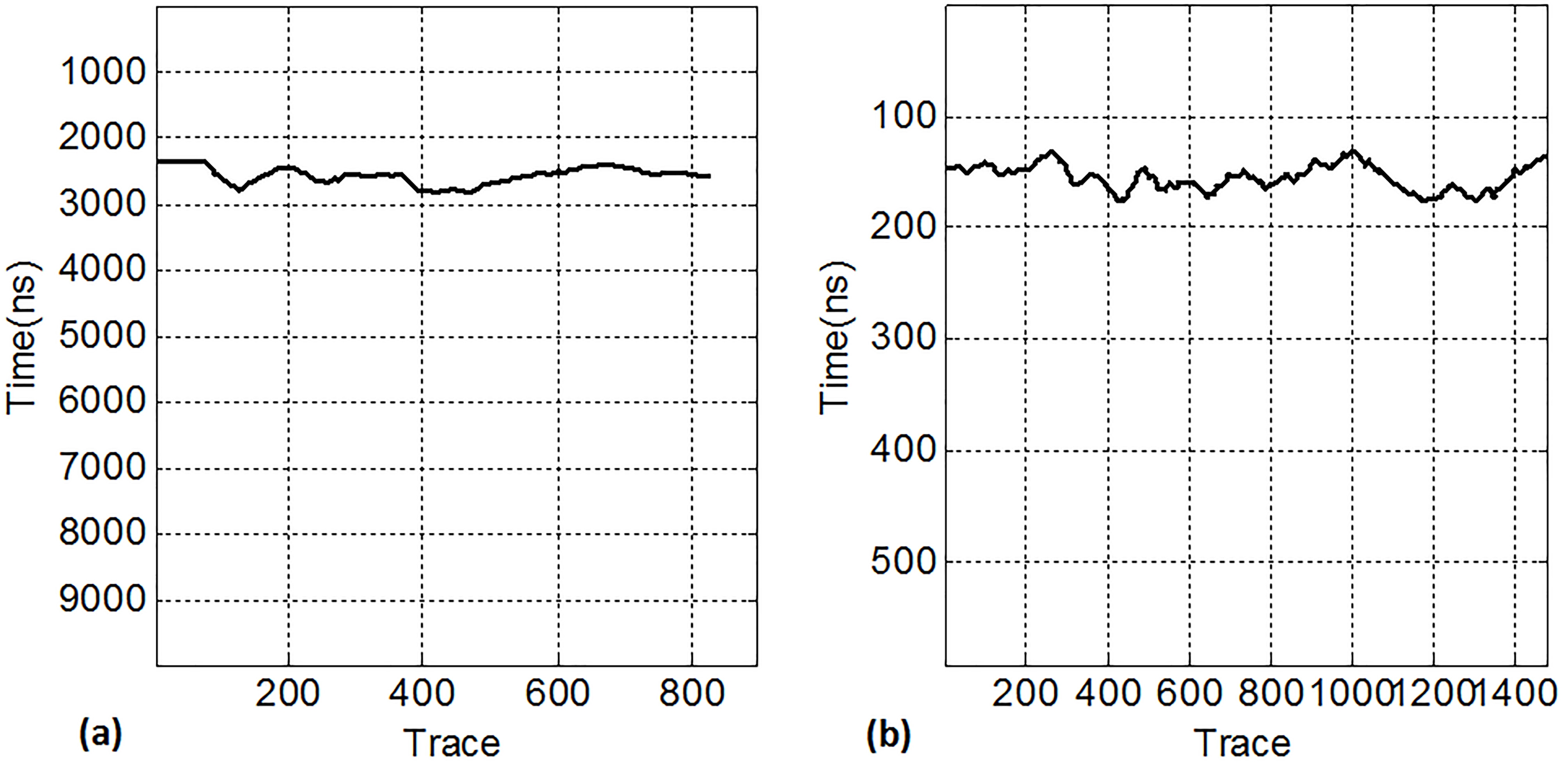Fig. 12 The estimated depth of the two channels. (a) The depth curve of Channel 1; (b) the depth curve of Channel 2.

## 5 Analysis and Discussion

The depths estimated by three methods are listed in Table 3.

Calibration parameters High-voltage-off data
Channel 1 60 MHz 152.4 m, 2610.4 ns 123.3 m, 2112.3 ns 136.9 m–165.5 m, 2344.7 ns–2834.2 ns
Channel 2 500 MHz 18.4 m, 185.5 ns 17.4 m, 176.1 ns 13.0 m–17.5 m, 131.0 ns–176.5 ns

Notes: In Channel 1, ε r is 6.6 and in Channel 2, ε r is 2.3.

Table 3 The Depths Estimated by Three Methods

The first method is based on the system calibration parameters and radar equation, which is a theoretical calculation method. Because some a priori assumptions, such as radio scattering sectional area, attenuation in the loss medium and dielectric constant, need to be assumed, the accuracy of assumptions will directly influence the final calculation results. Moreover, there must be some differences between the ground calibration environment and the in-situ detection environment, so unavoidable deviation exists in the results. In addition, the intensity of signal in the whole time delay of real data is larger than minimum detection power, thus it is not accurate when we use the minimum detection power as the threshold.

In the second method, we calculate the noise with the high-voltage-off data and replace the minimum detection power with the noise value, which is greater than what is used in the first method. However, using the radar equation inherently makes some assumptions, which will eventually influence the results.

In the third method, the radar equation and the ground calibration parameter are not involved and we estimate the depth only with the measured radar data. This method is based on the different correlation coefficient between the reflected echoes and noise to obtain the distance-varying penetrating depth along the profile of the rover. Actually, as the rover moves along the lunar surface, the depth should not be constant. Therefore, the CCM method is more consistent with the real situation than the traditional methods.

Different display ranges, namely dynamic range, are used to show the weak signal and eventually to verify the validity of CCM results. If the original display range is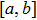, it means the maximum gray value of the figure is a and the minimum gray value is b. So when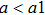and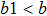, if we want to show a figure with the original range ofin a new display range of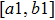, we need to find all gray values in the figure which are bigger than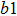and then change them to, and find all gray values which are less than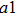and then change them to.

A comparison of the Channel 1 radar profile displayed in different ranges (−10 to 10, −1 to 1, −0.5 to 0.5, −0.2 to 0.2) with the associated depth curve is shown in Figure 13, in which the depth curve is red. A comparison of the Channel 2 radar profile is shown in Figure 14. The smaller the dynamic range is, the more details the figure will show. These figures demonstrate that the appearance of a signal above the red line indicates typical reflected echoes, and the appearance below the red line does not, which implies noise is dominant. Moreover, in the two figures, the trend in depth curve is very consistent with the trend in reflected echoes disappearing. However, regarding the 60 MHz data, because of the lower frequency, the lower depth resolution and the more interference caused by the roverʼs body, these data were worse than the 500 MHz data.Fig. 13 A comparison of Channel 1 radar profile displayed in different dynamic ranges with the depth curve.Fig. 14 A comparison of Channel 2 radar profile displayed in different dynamic ranges with the depth curve.

Figure 13 shows that the penetrating depth obtained by CCM is just the location where this interference disappears, which means the real penetrating depth of Channel 1 must be less than or equal to this depth. To be exact, the depth of Channel 1 obtained by CCM is the maximum possible penetrating depth, or ultimate penetrating depth. So finally, the results can be described in that the penetrating depth of Channel 2 is from 13.0 m to 17.5 m and the ultimate penetrating depth of Channel 1 is from 136.9 m to 165.5 m.

## 6 Conclusions

In this study, we analyze the penetrating depth of LPR with three methods, among which the first two methods utilize the radar equation and they are more inclined to be applied in theoretical calculations. The CCM, based on the different correlation between reflected echoes and noise to acquire the penetrating depth, is the main work of this study, which is first introduced and used for LPR data. Without a priori assumptions about the structure and dielectric properties of the Moon, CCM estimates the distribution of depth only using measured data. Through analysis of the results of three methods, CCM is verified to be an effective and practical method to estimate penetrating depth. The results indicate that the ultimate penetrating depth of Channel 1 (N0106 to N0207) ranges from 136.9 m to 165.5 m with a dielectric constant of 6.6, and the penetrating depth of Channel 2 (N0105 to N0208) ranges from 13.0 m to 17.5 m with a dielectric constant of 2.3.

However, it should be noted that the depth estimated by CCM is the boundary between reflected echoes and noise, but it does not mean that there is a real reflected layer at this depth. Especially for the Channel 1 data, the interference is strong enough to hide the subsurface reflected signal, so maybe the real penetrating depth is much shallower. Moreover, whether there are other useful echoes hiding in the noise and whether they can be extracted from the noise are controversial and need further study.

 Coppens F. 1985 Geophysical Prospecting 33 1212 Dai S. Su Y. Xiao Y. et al. 2014 RAA(Research in Astronomy and Astrophysics) 14 1642 Davis J. L. Annan A. P. 1989 Geophysical Prospecting 37 531 Fang G.-Y. Zhou B. Ji Y.-C. et al. 2014 RAA(Research in Astronomy and Astrophysics) 14 1607 Gelchinsky B. Shtivelman V. 1983 Geophysical Prospecting 31 915 Heiken G. H. Vaniman D. T. French B. M. 1991 Lunar Sourcebook — A User’S Guide to the Moon Cambridge Cambridge University Press Ling Z. Jolliff B. L. Wang A. et al. 2015 Nature Communications 6 Ono T. Kumamoto A. Nakagawa H. et al. 2009 Science 323 909 Su Y. Fang G.-Y. Feng J.-Q. et al. 2014 RAA(Research in Astronomy and Astrophysics) 14 1623 Tugnait J. K. 1993 IEEE Transactions on Signal Processing 41 549 Ulaby F. T. Moore R. K. Fung A. K. 2015 Microwave Remote Sensing Active and Passive London Addison-Wesley Publishing Company Zhang H.-B. Zheng L. Su Y. et al. 2014 RAA(Research in Astronomy and Astrophysics) 14 1633
Cite this article: Xing Shu-Guo, Su Yan, Feng Jian-Qing, Dai Shun, Xiao Yuan, Ding Chun-Yu, Li Chun-Lai. The penetrating depth analysis of Lunar Penetrating Radar onboard Chang’e-3 rover. Res. Astron. Astrophys. 2017; 5:046.

### Refbacks

• There are currently no refbacks.